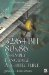Vector Scalar Addition and SubtractionScalar addition and subtraction of vectors are also a relative simple matter for vector math instructions to handle. Scalar math appears in one of two forms: either a single element processed within each vector, or one element is swizzled, shuffled, or splat (see Chapter 6, "Data Conversion") into each element position and applied to the other source vector. When this type instruction is not supported by a processor, the trick is to replicate the scalar so it appears as a second vector.

Listing 12-13: ...\chap12\vas3d\Vas3D.cppvoid vmp_VecAddScalar(vmp3DVector * const pvD,           const vmp3DVector * const pvA, float fScalar) {   pvD->x = pvA->x + fScalar;   pvD->y = pvA->y + fScalar;   pvD->z = pvA->z + fScalar; }Single-Precision Quad Vector Float Scalar Subtraction

Listing 12-14: ...\chap12\qvas3d\QVas3D.cppvoid vmp_VecSubScalar(vmp3DVector * const pvD,           const vmp3DVector * const pvA, float fScalar) {   pvD->x = pvA->x - fScalar;   pvD->y = pvA->y - fScalar;   pvD->z = pvA->z  fScalar; }Did that look strangely familiar? The big question now is, "How do we replicate a scalar to look like a vector since there tends not to be mirrored scalar math on processors?" Typically a processor will interpret a scalar calculation as the lowest (first) float being evaluated with a single scalar float. This is fine and dandy, but there are frequent times when a scalar needs to be replicated and summed to each element of a vector. So the next question is how do we do that?

With the 3DNow! instruction set it is easy. Since the processor is really a 64-bit half vector, the data is merely unpacked into the upper and lower 32 bits.

movd      mm2,fScalar     ; fScalar {0 s} punpckldq mm2,mm2         ; fScalar {s s}

Then it is just used twice, once with the upper 64 bits and then once with the lower 64 bits.

pfadd     mm0,mm2         ; {Ay+s Ax+s} pfadd     mm1,mm2         ; {Aw+s Az+s}

With the SSE instruction set it is almost as easy. The data is shuffled into all 32-bit floats.

movss    xmm1,fScalar            ; {0 0 0 s} shufps   xmm1,xmm1,00000000b     ; {s s s s}

Now the scalar is the same as the vector.

addps   xmm0,xmm1     ; {Aw+s Az+s Ay+s Ax+s}

Any questions?32/64-Bit 80x86 Assembly Language Architecture
ISBN: 1598220020
EAN: 2147483647
Year: 2003
Pages: 191

Similar book on Amazon